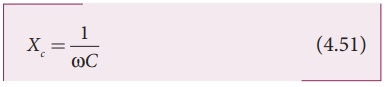Home | | Physics 12th Std | AC circuit containing only a capacitor

# AC circuit containing only a capacitor

Consider a circuit containing a capacitor of capacitance C connected across an alternating voltage source.

AC circuit containing only a capacitor

Consider a circuit containing a capacitor of capacitance C connected across an alternating voltage source (Figure 4.49). The alternating voltage is given byLet q be the instantaneous charge on the capacitor. The emf across the capacitor at that instant is q/C According to Kirchoffâ€™s loop rule,By the definition of current,Instantaneous value of current,where [Vm] / [1/CĎ‰] = Im , the peak value of the  alternating current. From equations (4.49) and (4.50), it is clear that current leads the applied voltage by Ď€/2 in a capacitive circuit. This is shown pictorially in Figure 4.50. The wave diagram for a capacitive circuit also shows that the current leads the applied voltage by 90Âş.

## Capacitive reactance XC

The peak value of current Im is given by Im = [Vm] / [1/CĎ‰].  . Let us compare this equation with Im = Vm/R from resistive circuit. The quantity 1/ CĎ‰  plays the same role as the resistance R in resistive circuit. This is the resistance offered by the capacitor, called  capacitive reactance (XC). It measured in ohm.The capacitive reactance (XC) varies inversely as the frequency. For a steady current, f = 0.Thus a capacitive circuit offers infinite resistance to the steady current. So that steady current cannot flow through the capacitor.EXAMPLE 4.21

A capacitor of capacitance 102/Ď€ ÂµF is connected across a 220 V, 50 Hz A.C. mains. Calculate the capacitive reactance, RMS value of current and write down the equations of voltage and current.

SolutionTags : Phasor diagram, Circuit Diagram, Formula, Solved Example Problems | Alternating Current (AC) , 12th Physics : Electromagnetic Induction and Alternating Current
Study Material, Lecturing Notes, Assignment, Reference, Wiki description explanation, brief detail
12th Physics : Electromagnetic Induction and Alternating Current : AC circuit containing only a capacitor | Phasor diagram, Circuit Diagram, Formula, Solved Example Problems | Alternating Current (AC)